### Displayed Output

Subsections:

#### Number of Variable Levels Table

If you specify the NLEVELS option in the PROC FREQ statement, PROC FREQ displays the Number of Variable Levels table. This table provides the number of levels for all variables named in the TABLES statements. PROC FREQ determines the variable levels from the formatted variable values. See Grouping with Formats for details. The Number of Variable Levels table contains the following information:

• Variable name

• Levels, which is the total number of levels of the variable

• Number of Nonmissing Levels, if there are missing levels for any of the variables

• Number of Missing Levels, if there are missing levels for any of the variables

#### One-Way Frequency Tables

PROC FREQ displays one-way frequency tables for all one-way table requests in the TABLES statements, unless you specify the NOPRINT option in the PROC FREQ statement or the NOPRINT option in the TABLES statement. For a one-way table showing the frequency distribution of a single variable, PROC FREQ displays the name of the variable and its values. For each variable value or level, PROC FREQ displays the following information:

• Frequency count, which is the number of observations in the level

• Test Frequency count, if you specify the CHISQ and TESTF= options to request a chi-square goodness-of-fit test for specified frequencies

• Percent, which is the percentage of the total number of observations. (The NOPERCENT option suppresses this information.)

• Test Percent, if you specify the CHISQ and TESTP= options to request a chi-square goodness-of-fit test for specified percents. (The NOPERCENT option suppresses this information.)

• Cumulative Frequency count, which is the sum of the frequency counts for that level and all other levels listed above it in the table. The last cumulative frequency is the total number of nonmissing observations. (The NOCUM option suppresses this information.)

• Cumulative Percent, which is the percentage of the total number of observations in that level and in all other levels listed above it in the table. (The NOCUM or the NOPERCENT option suppresses this information.)

The one-way table also displays the Frequency Missing, which is the number of observations with missing values.

#### Statistics for One-Way Frequency Tables

For one-way tables, two statistical options are available in the TABLES statement. The CHISQ option provides a chi-square goodness-of-fit test, and the BINOMIAL option provides binomial proportion statistics and tests. PROC FREQ displays the following information, unless you specify the NOPRINT option in the PROC FREQ statement:

• If you specify the CHISQ option for a one-way table, PROC FREQ provides a chi-square goodness-of-fit test, displaying the Chi-Square statistic, the degrees of freedom (DF), and the probability value (Pr > ChiSq). If you specify the CHISQ option in the EXACT statement, PROC FREQ also displays the exact probability value for this test. If you specify the POINT option with the CHISQ option in the EXACT statement, PROC FREQ displays the exact point probability for the test statistic. If you specify the MIDP option in the EXACT statement, PROC FREQ displays the exact mid p-value for the chi-square test.

• If you specify the BINOMIAL option for a one-way table, PROC FREQ displays the estimate of the binomial Proportion, which is the proportion of observations in the first class listed in the one-way table. PROC FREQ also displays the asymptotic standard error (ASE) and the asymptotic (Wald) and exact (Clopper-Pearson) confidence limits by default. For the binomial proportion test, PROC FREQ displays the asymptotic standard error under the null hypothesis (ASE Under H0), the standardized test statistic (Z), and the one-sided and two-sided probability values.

If you specify the BINOMIAL option in the EXACT statement, PROC FREQ also displays the exact one-sided and two-sided probability values for this test. If you specify the POINT option with the BINOMIAL option in the EXACT statement, PROC FREQ displays the exact point probability for the test. If you specify the MIDP option in the EXACT statement, PROC FREQ displays the exact mid p-value for the binomial proportion test.

• If you request additional binomial confidence limits by specifying binomial-options, PROC FREQ provides a table that displays the lower and upper confidence limits for each type that you request. In addition to the Wald and exact (Clopper-Pearson) confidence limits, you can request Agresti-Coull, Jeffreys, and Wilson (score) confidence limits for the binomial proportion.

• If you request a binomial noninferiority or superiority test by specifying the NONINF or SUP binomial-option, PROC FREQ displays a Noninferiority Analysis or Superiority Analysis table that contains the following information: the binomial Proportion, the test ASE (under H0 or Sample), the test statistic Z, the probability value, the noninferiority or superiority limit, and the test confidence limits. If you specify the BINOMIAL option in the EXACT statement, PROC FREQ also provides the exact probability value for the test, and exact test confidence limits.

• If you request a binomial equivalence test by specifying the EQUIV binomial-option, PROC FREQ displays an Equivalence Analysis table that contains the following information: binomial Proportion and the test ASE (under H0 or Sample). PROC FREQ displays two one-sided tests (TOST) for equivalence, which include test statistics (Z) and probability values for the Lower and Upper tests, together with the Overall probability value. PROC FREQ also displays the equivalence limits and the test-based confidence limits. If you specify the BINOMIAL option in the EXACT statement, PROC FREQ provides exact probability values for the TOST and exact test-based confidence limits.

#### Two-Way and Multiway Tables

PROC FREQ displays all multiway table requests in the TABLES statements, unless you specify the NOPRINT option in the PROC FREQ statement or the NOPRINT option in the TABLES statement.

For two-way to multiway crosstabulation tables, the values of the last variable in the table request form the table columns. The values of the next-to-last variable form the rows. Each level (or combination of levels) of the other variables forms one stratum.

There are three ways to display multiway tables in PROC FREQ. By default, PROC FREQ displays multiway tables as separate two-way crosstabulation tables for each stratum of the multiway table. Also by default, PROC FREQ displays these two-way crosstabulation tables in table cell format. Alternatively, if you specify the CROSSLIST option, PROC FREQ displays the two-way crosstabulation tables in ODS column format. If you specify the LIST option, PROC FREQ displays multiway tables in list format, which presents the entire multiway crosstabulation in a single table.

##### Crosstabulation Tables

By default, PROC FREQ displays two-way crosstabulation tables in table cell format. The row variable values are listed down the side of the table, the column variable values are listed across the top of the table, and each row and column variable level combination forms a table cell.

Each cell of a crosstabulation table can contain the following information:

• Frequency, which is the number of observations in the table cell. (The NOFREQ option suppresses this information.)

• Expected frequency under the hypothesis of independence, if you specify the EXPECTED option

• Deviation of the cell frequency from the expected value, if you specify the DEVIATION option

• Cell Chi-Square, which is the cell’s contribution to the total chi-square statistic, if you specify the CELLCHI2 option

• Tot Pct, which is the cell’s percentage of the total multiway table frequency, for n-way tables when n > 2, if you specify the TOTPCT option

• Percent, which is the cell’s percentage of the total (two-way table) frequency. (The NOPERCENT option suppresses this information.)

• Row Pct, or the row percentage, which is the cell’s percentage of the total frequency for its row. (The NOROW option suppresses this information.)

• Col Pct, or column percentage, which is the cell’s percentage of the total frequency for its column. (The NOCOL option suppresses this information.)

• Cumulative Col%, or cumulative column percentage, if you specify the CUMCOL option

The table also displays the Frequency Missing, which is the number of observations with missing values.

##### CROSSLIST Tables

If you specify the CROSSLIST option, PROC FREQ displays two-way crosstabulation tables in ODS column format. The CROSSLIST column format is different from the default crosstabulation table cell format, but the CROSSLIST table provides the same information (frequencies, percentages, and other statistics) as the default crosstabulation table.

In the CROSSLIST table format, the rows of the display correspond to the crosstabulation table cells, and the columns of the display correspond to descriptive statistics such as frequencies and percentages. Each table cell is identified by the values of its TABLES row and column variable levels, with all column variable levels listed within each row variable level. The CROSSLIST table also provides row totals, column totals, and overall table totals.

For a crosstabulation table in CROSSLIST format, PROC FREQ displays the following information:

• the row variable name and values

• the column variable name and values

• Frequency, which is the number of observations in the table cell. (The NOFREQ option suppresses this information.)

• Expected cell frequency under the hypothesis of independence, if you specify the EXPECTED option

• Deviation of the cell frequency from the expected value, if you specify the DEVIATION option

• Standardized Residual, if you specify the CROSSLIST(STDRES) option

• Pearson Residual, if you specify the CROSSLIST(PEARSONRES) option

• Cell Chi-Square, which is the cell’s contribution to the total chi-square statistic, if you specify the CELLCHI2 option

• Total Percent, which is the cell’s percentage of the total multiway table frequency, for n-way tables when n > 2, if you specify the TOTPCT option

• Percent, which is the cell’s percentage of the total (two-way table) frequency. (The NOPERCENT option suppresses this information.)

• Row Percent, which is the cell’s percentage of the total frequency for its row. (The NOROW option suppresses this information.)

• Column Percent, the cell’s percentage of the total frequency for its column. (The NOCOL option suppresses this information.)

The table also displays the Frequency Missing, which is the number of observations with missing values.

##### LIST Tables

If you specify the LIST option in the TABLES statement, PROC FREQ displays multiway tables in a list format rather than as crosstabulation tables. The LIST option displays the entire multiway table in one table, instead of displaying a separate two-way table for each stratum. The LIST option is not available when you also request statistical options. Unlike the default crosstabulation output, the LIST output does not display row percentages, column percentages, and optional information such as expected frequencies and cell chi-squares.

For a multiway table in list format, PROC FREQ displays the following information:

• the variable names and values

• Frequency, which is the number of observations in the level (with the indicated variable values)

• Percent, which is the level’s percentage of the total number of observations. (The NOPERCENT option suppresses this information.)

• Cumulative Frequency, which is the accumulated frequency of the level and all other levels listed above it in the table. The last cumulative frequency in the table is the total number of nonmissing observations. (The NOCUM option suppresses this information.)

• Cumulative Percent, which is the accumulated percentage of the level and all other levels listed above it in the table. (The NOCUM or the NOPERCENT option suppresses this information.)

The table also displays the Frequency Missing, which is the number of observations with missing values.

#### Statistics for Two-Way and Multiway Tables

PROC FREQ computes statistical tests and measures for crosstabulation tables, depending on which statements and options you specify. You can suppress the display of these results by specifying the NOPRINT option in the PROC FREQ statement. With any of the following information, PROC FREQ also displays the Sample Size and the Frequency Missing.

• If you specify the SCOROUT option in the TABLES statement, PROC FREQ displays the Row Scores and Column Scores that it uses for statistical computations. The Row Scores table displays the row variable values and the Score corresponding to each value. The Column Scores table displays the column variable values and the corresponding Scores. PROC FREQ also identifies the score type used to compute the row and column scores. You can specify the score type with the SCORES= option in the TABLES statement.

• If you specify the CHISQ option, PROC FREQ displays the following statistics for each two-way table: Pearson Chi-Square, Likelihood Ratio Chi-Square, Continuity-Adjusted Chi-Square (fortables), Mantel-Haenszel Chi-Square, the Phi Coefficient, the Contingency Coefficient, and Cramér’s V. For each test statistic, PROC FREQ also displays the degrees of freedom (DF) and the probability value (Prob).

• If you specify the CHISQ option fortables, PROC FREQ also displays Fisher’s exact test. The test output includes the cell (1,1) frequency (F), the exact left-sided and right-sided probability values, the table probability (P), and the exact two-sided probability value. If you specify the POINT option in the EXACT statement, PROC FREQ displays the exact point probability for Fisher’s exact test. If you specify the MIDP option in the EXACT statement, PROC FREQ displays the Mid p-Value for the test.

• If you specify the FISHER option in the TABLES statement (or, equivalently, the FISHER option in the EXACT statement), PROC FREQ displays Fisher’s exact test for tables larger than. The test output includes the table probability (P) and the probability value. If you specify the POINT option in the EXACT statement, PROC FREQ displays the exact point probability for Fisher’s exact test. If you specify the MIDP option in the EXACT statement, PROC FREQ displays the Mid p-Value for the test.

• If you specify the PCHI, LRCHI, or MHCHI option in the EXACT statement, PROC FREQ displays the corresponding exact test: Pearson Chi-Square, Likelihood Ratio Chi-Square, or Mantel-Haenszel Chi-Square, respectively. The test output includes the test statistic, the degrees of freedom (DF), and the asymptotic and exact probability values. If you also specify the POINT option in the EXACT statement, PROC FREQ displays the point probability for each exact test requested. If you specify the MIDP option in the EXACT statement, PROC FREQ displays the exact mid p-value for each test. If you specify the CHISQ option in the EXACT statement, PROC FREQ displays exact probability values for all three of these chi-square tests.

• If you specify the MEASURES option, PROC FREQ displays the following statistics and their asymptotic standard errors (ASE) for each two-way table: Gamma, Kendall’s Tau-b, Stuart’s Tau-c, Somers’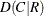, Somers’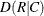, Pearson Correlation, Spearman Correlation, Lambda Asymmetric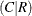, Lambda Asymmetric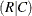, Lambda Symmetric, Uncertainty Coefficient, Uncertainty Coefficient, and Uncertainty Coefficient Symmetric. If you specify the CL option, PROC FREQ also displays confidence limits for these measures.

• If you specify the PLCORR option, PROC FREQ displays the tetrachoric correlation fortables or the polychoric correlation for larger tables. In addition, PROC FREQ displays the MEASURES output listed earlier, even if you do not also specify the MEASURES option.

• If you specify the GAMMA, KENTB, STUTC, SMDCR, SMDRC, PCORR, or SCORR option in the TEST statement, PROC FREQ displays asymptotic tests for Gamma, Kendall’s Tau-b, Stuart’s Tau-c, Somers’, Somers’, the Pearson Correlation, or the Spearman Correlation, respectively. If you specify the MEASURES option in the TEST statement, PROC FREQ displays all these asymptotic tests. The test output includes the statistic, its asymptotic standard error (ASE), Confidence Limits, the ASE under the null hypothesis H0, the standardized test statistic (Z), and the one-sided and two-sided probability values.

• If you specify the KENTB, STUTC, SMDCR, SMDRC, PCORR, or SCORR option in the EXACT statement, PROC FREQ displays asymptotic and exact tests for the corresponding measure of association: Kendall’s Tau-b, Stuart’s Tau-c, Somers’, Somers’, the Pearson Correlation, or the Spearman correlation, respectively. The test output includes the correlation, its asymptotic standard error (ASE), Confidence Limits, the ASE under the null hypothesis H0, the standardized test statistic (Z), and the asymptotic and exact one-sided and two-sided probability values. If you also specify the POINT option in the EXACT statement, PROC FREQ displays the point probability for each exact test requested. If you specify the MIDP option in the EXACT statement, PROC FREQ displays the exact Mid p-Value for each test.

• If you specify the RISKDIFF option fortables, PROC FREQ displays the Column 1 and Column 2 Risk Estimates. For each column, PROC FREQ displays the Row 1 Risk, Row 2 Risk, Total Risk, and Risk Difference, together with their asymptotic standard errors (ASE) and Asymptotic Confidence Limits. PROC FREQ also displays Exact Confidence Limits for the Row 1 Risk, Row 2 Risk, and Total Risk. If you specify the RISKDIFF option in the EXACT statement, PROC FREQ provides unconditional Exact Confidence Limits for the Risk Difference. You can suppress this table by specifying the RISKDIFF(NORISKS) option.

• If you specify the RISKDIFF(CL=) option fortables, PROC FREQ displays the Confidence Limits for the Proportion (Risk) Difference table, which includes the Lower and Upper Confidence Limits for each confidence limit Type that you request (Exact, Hauck-Anderson, Miettinen-Nurminen score, Newcombe, or Wald).

• If you request a noninferiority or superiority test for the proportion difference (RISKDIFF) by specifying the NONINF or SUP riskdiff-option, and if you specify METHOD=HA (Hauck-Anderson), METHOD=FM (Farrington-Manning score), or METHOD=WALD (Wald), PROC FREQ displays the following information: the Proportion Difference, the test ASE (H0, Sample, Sample H-A, or FM, depending on the method you specify), the test statistic Z, the probability value, the Noninferiority or Superiority Limit, and the test-based Confidence Limits. If you specify METHOD=NEWCOMBE (Newcombe), PROC FREQ displays the Proportion Difference, the Noninferiority or Superiority Limit, and the Newcombe Confidence Limits.

• If you request an equivalence test for the proportion difference (RISKDIFF) by specifying the EQUIV riskdiff-option, and if you specify METHOD=HA (Hauck-Anderson), METHOD=FM (Farrington-Manning score), or METHOD=WALD (Wald), PROC FREQ displays the following information: the Proportion Difference and the test ASE (H0, Sample, Sample H-A, or FM, depending on the method you specify). PROC FREQ displays a two one-sided test (TOST) for equivalence, which includes test statistics (Z) and probability values for the Lower and Upper tests, together with the Overall probability value. PROC FREQ also displays the Equivalence Limits and the test-based Confidence Limits. If you specify METHOD=NEWCOMBE (Newcombe), PROC FREQ displays the Proportion Difference, the Equivalence Limits, and the Newcombe Confidence Limits.

• If you request an equality test for the proportion difference (RISKDIFF) by specifying the EQUAL riskdiff-option, PROC FREQ displays the following information: the Proportion Difference and the test ASE (H0 or Sample), the test statistic Z, the One-Sided probability value (Pr > Z or Pr < Z), and the Two-Sided probability value, Pr > |Z|.

• If you specify the MEASURES option or the RELRISK option fortables, PROC FREQ displays the Odds Ratio and Relative Risks table, which includes the following statistics with their confidence limits: Odds Ratio, Relative Risk (Column 1), and Relative Risk (Column 2). If you specify the OR option in the EXACT statement, PROC FREQ also displays the Exact Confidence Limits for the Odds Ratio table If you specify the RELRISK option in the EXACT statement, PROC FREQ displays the Exact Confidence Limits for the Relative Risk table.

• If you specify the OR(CL=) option fortables, PROC FREQ displays the Confidence Limits for the Odds Ratio table, which includes the Lower and Upper Confidence Limits for each confidence limit Type that you request (Exact, Score, or Wald).

• If you specify the TREND option, PROC FREQ displays the Cochran-Armitage Trend Test for tables that are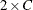or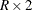. For this test, PROC FREQ gives the Statistic (Z) and the one-sided and two-sided probability values. If you specify the TREND option in the EXACT statement, PROC FREQ also displays the exact one-sided and two-sided probability values for this test. If you specify the POINT option with the TREND option in the EXACT statement, PROC FREQ displays the exact point probability for the test statistic. If you specify the MIDP option in the EXACT statement, PROC FREQ displays the exact Mid p-Value for the trend test.

• If you specify the JT option, PROC FREQ displays the Jonckheere-Terpstra Test, showing the Statistic (JT), the standardized test statistic (Z), and the one-sided and two-sided probability values. If you specify the JT option in the EXACT statement, PROC FREQ also displays the exact one-sided and two-sided probability values for this test. If you specify the POINT option with the JT option in the EXACT statement, PROC FREQ displays the exact point probability for the test statistic. If you specify the MIDP option in the EXACT statement, PROC FREQ displays the exact Mid p-Value for the Jonckheere-Terpstra test.

• If you specify the AGREE(PRINTKWTS) option, PROC FREQ displays the Kappa Coefficient Weights for square tables of dimension greater than 2.

• If you specify the AGREE option, for two-way tables PROC FREQ displays McNemar’s Test and the Simple Kappa Coefficient fortables. For square tables larger than, PROC FREQ displays Bowker’s Test of Symmetry, the Simple Kappa Coefficient, and the Weighted Kappa Coefficient. For McNemar’s Test and Bowker’s Test of Symmetry, PROC FREQ displays the Statistic (S), the degrees of freedom (DF), and For the simple and weighted kappa coefficients, PROC FREQ displays the kappa values, asymptotic standard errors (ASE), and Confidence Limits. the probability value (Pr > S).

If you specify the MCNEM option in the EXACT statement, PROC FREQ also displays the exact probability value for McNemar’s test. If you specify the POINT option with the MCNEM option in the EXACT statement, PROC FREQ displays the exact point probability for the test statistic. If you specify the MIDP option in the EXACT statement, PROC FREQ displays the Exact Mid p-Value for McNemar’s test.

• If you specify the KAPPA or WTKAP option in the TEST statement, PROC FREQ displays asymptotic tests for the simple kappa coefficient or the weighted kappa coefficient, respectively. If you specify the AGREE option in the TEST statement, PROC FREQ displays both these asymptotic tests. The test output includes the kappa coefficient, its asymptotic standard error (ASE), Confidence Limits, the ASE under the null hypothesis H0, the standardized test statistic (Z), and the one-sided and two-sided probability values.

• If you specify the KAPPA or WTKAP option in the EXACT statement, PROC FREQ displays asymptotic and exact tests for the simple kappa coefficient or the weighted kappa coefficient, respectively. The test output includes the kappa coefficient, its asymptotic standard error (ASE), Confidence Limits, the ASE under the null hypothesis H0, the standardized test statistic (Z), and the asymptotic and exact one-sided and two-sided probability values. If you specify the POINT option in the EXACT statement, PROC FREQ displays the point probability for each exact test requested. If you specify the MIDP option in the EXACT statement, PROC FREQ displays the exact Mid p-Value for each exact test.

• If you specify the MC option in the EXACT statement, PROC FREQ displays Monte Carlo estimates for all exact p-values requested by statistic-options in the EXACT statement. The Monte Carlo output includes the p-value Estimate, its Confidence Limits, the Number of Samples used to compute the Monte Carlo estimate, and the Initial Seed for random number generation.

• If you specify the AGREE option for a multiway square table, PROC FREQ displays Overall Simple and Weighted Kappa Coefficients, with their asymptotic standard errors (ASE) and Confidence Limits. PROC FREQ also displays Tests for Equal Kappa Coefficients, giving the Chi-Squares, degrees of freedom (DF), and probability values (Pr > ChiSq) for the Simple Kappa and Weighted Kappa. For multiple strata oftables, PROC FREQ displays Cochran’s Q, giving the Statistic (Q), the degrees of freedom (DF), and the probability value (Pr > Q).

• If you specify the RISKDIFF(COMMON) option for a multiwaytable, PROC FREQ displays the Common Proportion (Risk) Difference table, which includes the following information: Method (Mantel-Haenszel or Summary Score), Value of the common risk difference, Standard Error, and Confidence Limits.

• If you specify the CMH option, PROC FREQ displays Cochran-Mantel-Haenszel Statistics for the following three alternative hypotheses: Nonzero Correlation, Row Mean Scores Differ (ANOVA Statistic), and General Association. For each of these statistics, PROC FREQ gives the degrees of freedom (DF) and the probability value (Prob). If you specify the MANTELFLEISS option, PROC FREQ displays the Mantel-Fleiss Criterion fortables. Fortables, PROC FREQ also displays Estimates of the Common Relative Risk for Case-Control and Cohort studies, together with their confidence limits. These include both Mantel-Haenszel and Logit stratum-adjusted estimates of the common Odds Ratio, Column 1 Relative Risk, and Column 2 Relative Risk. Also fortables, PROC FREQ displays the Breslow-Day Test for Homogeneity of the Odds Ratios. For this test, PROC FREQ gives the Chi-Square, the degrees of freedom (DF), and the probability value (Pr > ChiSq).

• If you specify the CMH option in the TABLES statement and also specify the COMOR option in the EXACT statement for a multiwaytable, PROC FREQ displays exact confidence limits for the Common Odds Ratio. PROC FREQ also displays the Exact Test of H0: Common Odds Ratio = 1. The test output includes the Cell (1,1) Sum (S), Mean of S Under H0, One-sided Pr <= S, and Point Pr = S. PROC FREQ also provides exact two-sided probability values for the test, computed according to the following three methods: 2 * One-sided, Sum of probabilities <= Point probability, and Pr >= |S - Mean|. If you specify the MIDP option in the EXACT statement, PROC FREQ provides the exact Mid p-Value for the common odds ratio test.

• If you specify the CMH option in the TABLES statement and also specify the EQOR option in the EXACT statement for a multiwaytable, PROC FREQ computes Zelen’s exact test for equal odds ratios. PROC FREQ displays Zelen’s test along with the asymptotic Breslow-Day test produced by the CMH option. PROC FREQ displays the test statistic, Zelen’s Exact Test (P), and the probability value, Exact Pr <= P.

• If you specify the GAILSIMON option in the TABLES statement for a multiwaytables, PROC FREQ displays the Gail-Simon test for qualitative interactions. The display include the following statistics and their p-values: Q+ (Positive Risk Differences), Q- (Negative Risk Differences), and Q (Two-Sided).